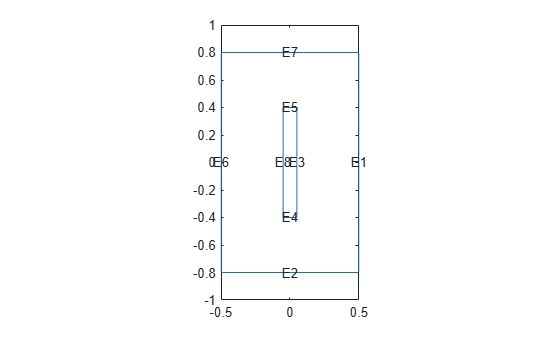linearizeOutput

Specify outputs of linearized model

Description

example

linearizeOutput(model,RegionType,RegionID) adds all degrees of freedom (DoFs) associated with the region defined by RegionType and RegionID to the output vector of the linearized model. For 3-D structural models, linearizeOutput adds all x-coordinates first, then all y-coordinates, then all z-coordinates. In the linearized model sys, use sys.OutputGroup to locate the sections associated with each coordinate.

Use linearizeInput and linearizeOutput together with the linearize function to extract sparse linear models from structural and thermal models.

example

linearizeOutput(model,RegionType,RegionID,'Component',xyz) specifies which of the coordinates to include.

output = linearizeOutput(___) returns a structure array output with the linearization output description. Use this syntax with any of the previous arguments.

Examples

collapse all

Specify the regions of a 2-D thermal model for which linearize extracts sparse linear models used in Control System Toolbox™.

Create a transient thermal model.

thermalmodel = createpde('thermal','transient');

Add the block geometry to the thermal model by using the geometryFromEdges function. The geometry description file for this problem is called crackg.m.

geometryFromEdges(thermalmodel,@crackg);

Plot the geometry, displaying edge labels.

pdegplot(thermalmodel,'EdgeLabels','on')
ylim([-1,1])
axis equalGenerate a mesh.

generateMesh(thermalmodel);

Specify the thermal conductivity, mass density, and specific heat of the material.

thermalProperties(thermalmodel,'ThermalConductivity',1, ...
'MassDensity',1, ...
'SpecificHeat',1);

Specify the temperature on the left edge as 100, and constant heat flow to the exterior through the right edge as -10. Add a unique label to each boundary condition.

thermalBC(thermalmodel,'Edge',6,'Temperature',100,'Label','TempBC');
thermalBC(thermalmodel,'Edge',1,'HeatFlux',-10,'Label','FluxBC');

Specify that the entire geometry generates heat and add a unique label to this assignment.

internalHeatSource(thermalmodel,25,'Label','HeatSource');

Set an initial value of 0 for the temperature.

thermalIC(thermalmodel,0);

Call the linearizeInput function with the previously defined labels for the boundary conditions and the internal heat source to set the inputs for the linearize function. Add one label per function call.

linearizeInput(thermalmodel,'HeatSource');
linearizeInput(thermalmodel,'TempBC');
linearizeInput(thermalmodel,'FluxBC');

Call the linearizeOutput function to specify the regions for which you want linearize to extract sparse linear models. Specify one region per function call.

linearizeOutput(thermalmodel,'Edge',2)
ans = struct with fields:
RegionType: 'Edge'
RegionID: 2

Specify which of the x-, y-, and z- coordinates to include in a linearized model.

Create a structural transient analysis model.

structuralmodel = createpde('structural','transient-solid');

Import and plot the tuning fork geometry.

importGeometry(structuralmodel,'TuningFork.stl');
pdegplot(structuralmodel)Generate a mesh.

generateMesh(structuralmodel,'Hmax',0.005);

Specify the Young's modulus, Poisson's ratio, and mass density to model linear elastic material behavior. Specify all physical properties in consistent units.

structuralProperties(structuralmodel,'YoungsModulus',210E9, ...
'PoissonsRatio',0.3, ...
'MassDensity',8000);

Identify faces for applying boundary constraints and loads by plotting the geometry with the face labels.

figure('units','normalized','outerposition',[0 0 1 1])
pdegplot(structuralmodel,'FaceLabels','on')
view(-50,15)
title 'Geometry with Face Labels'Impose sufficient boundary constraints to prevent rigid body motion under applied loading. Typically, you hold a tuning fork by hand or mount it on a table. As a simplified approximation of this boundary condition, fix a region near the intersection of the tines and the handle (faces 21 and 22).

structuralBC(structuralmodel,'Face',[21,22],'Constraint','fixed');

Specify the pressure loading on a tine as a short rectangular pressure pulse.

'Pressure',5E6, ...
'EndTime',1e-3, ...
'Label','Pressure');

Specify acceleration due to gravity as a body load.

'GravitationalAcceleration',[0 0 -1], ...
'Label','Gravity');

Create inputs for gravity and the pressure pulse on tuning fork.

linearizeInput(structuralmodel,'Gravity');
linearizeInput(structuralmodel,'Pressure');

Measure the y-displacement of face 12 and x-displacement of face 6.

linearizeOutput(structuralmodel,'Face',12,'Component','y')
ans = struct with fields:
RegionType: 'Face'
RegionID: 12
Component: 'y'

linearizeOutput(structuralmodel,'Face',6,'Component','x')
ans = struct with fields:
RegionType: 'Face'
RegionID: 6
Component: 'x'

Input Arguments

collapse all

Structural or linear thermal model, specified as a StructuralModel object or a ThermalModel object. The linearize function does not support nonlinear thermal analysis.

Geometric region type, specified as 'Cell' (for a 3-D model only), 'Face', 'Edge', or 'Vertex'.

Data Types: char

Geometric region ID, specified as a positive integer. Find the region IDs by using pdegplot with the 'CellLabels', 'FaceLabels', 'EdgeLabels', or 'VertexLabels' value set to 'on'.

Data Types: double

Coordinates to include, specified as a character vector or a string of x-, y-, and z-coordinates to include.

Example: linearizeOutput(pdemodel,'Face',10,'Component','xz') selects the x and z DoFs for face 10

Data Types: char | string

Output Arguments

collapse all

Linearization output description, returned as a structure array.